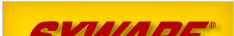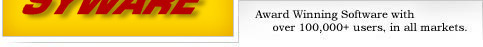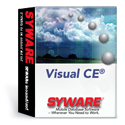mobile databases, mobile forms, and mobile synchronization … where you need to work+ Solutions + Products + Support + Purchase + Company + Contact# Numeric FunctionsABS(numeric_exp)
Returns the absolute value of numeric_exp.

ACOS(float_exp)
Returns the arccosine of float_exp as an angle, expressed in radians.

ASIN(float_exp)
Returns the arcsine of float_exp as an angle, expressed in radians.

ATAN(float_exp)
Returns the arctangent of float_exp as an angle, expressed in radians.

ATAN2(float_exp1, float_exp2)
Returns the arctangent of the x and y coordinates, specified by float_exp1 and float_exp2, respectively, as an angle, expressed in radians.

CEILING(numeric_exp)
Returns the smallest integer greater than or equal to numeric_exp. The return value is of the same data type as the input parameter.

COS(float_exp)
Returns the cosine of float_exp, where float_exp is an angle expressed in radians.

COUNT
Returns number of records.

COT(float_exp)
Returns the cotangent of float_exp, where float_exp is an angle expressed in radians.

DEGREES(numeric_exp)
Returns the number of degrees converted from numeric_exp radians.

EXP(float_exp)
Returns the exponential value of float_exp.

FLOOR(numeric_exp)
Returns the largest integer less than or equal to numeric_exp. The return value is of the same data type as the input parameter.

LOG(float_exp)
Returns the natural logarithm of float_exp.

LOG10(float_exp)
Returns the base 10 logarithm of float_exp.

MAX(expression)
Report CE only. Returns maximum value of expression.

MIN(expression)
Report CE only. Returns minimum value of expression.

MOD(integer_exp1, integer_exp2)
Returns the remainder (modulus) of integer_exp1 divided by integer_exp2.

PAGE
Report CE only. Returns current page number.

PI
Returns the constant value of pi as a floating-point value.

POWER(numeric_exp_1, numeric_exp_2)
Returns the value of numeric_exp_1 to the power of numeric_exp_2

Returns the number of radians converted from numeric_exp degrees.

RAND
Returns a random floating-point value.

ROUND(numeric_exp, integer_exp)
Returns numeric_exp rounded to integer_exp places right of the decimal point. If integer_exp is negative, numeric_exp is rounded to ABS(integer_exp) places to the left of the decimal point.

SIGN(numeric_exp)
Returns an indicator of the sign of numeric_exp. If numeric_exp is less than zero, -1 is returned. If numeric_exp equals zero, 0 is returned. If numeric_exp is greater than zero, 1 is returned.

SIN(float_exp)
Returns the sine of float_exp, where float_exp is an angle expressed in radians.

SQRT(float_exp)
Returns the square root of float_exp.

SUM(expression)
Report CE only. Returns sum of expression.

TAN(float_exp)
Returns the tangent of float_exp, where float_exp is an angle expressed in radians.

TRUNCATE(numeric_exp, integer_exp)
Returns numeric_exp truncated to integer_exp places right of the decimal point. If integer_exp is negative, numeric_exp is truncated to ABS(integer_exp) places to the left of the decimal point.